Introduction to Categorical Data Analysis Procedures
 Stratified Simple Random Sampling: Multiple Populations

Suppose you take two simple random samples, 50 men and 50 women, and ask the same question as before. You are now sampling two different populations that may have different response probabilities. The data can be tabulated as shown in Table 8.2.

Table 8.2 Two-Way Contingency Table: Sex by Color

Favorite Color

Sex

Red

Blue

Green

Total

Male

30

10

10

50

Female

20

10

20

50

Total

50

20

30

100

Note that the row marginal totals (50, 50) of the contingency table are fixed by the sampling design, but the column marginal totals (50, 20, 30) are random. There are six probabilities of interest for this table, and they are estimated by the sample proportions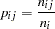where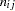denotes the frequency for theth population and theth response and
is the total frequency for theth population. For this contingency table, the sample proportions are shown in Table 8.3.

Table 8.3 Table of Sample Proportions by Sex

Favorite Color

Sex

Red

Blue

Green

Total

Male

0.60

0.20

0.20

1.00

Female

0.40

0.20

0.40

1.00

The probability distribution of the six frequencies is the product multinomial distribution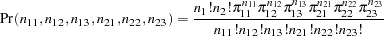where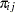is the true probability of observing theth response level in theth population. The product multinomial distribution is simply the product of two or more individual multinomial distributions since the populations are independent. This distribution can be generalized to any number of populations and response levels.

Stratified simple random sampling is the type of sampling required by the modeling procedures when there is more than one population. The product multinomial distribution is used to estimate a probability vector and its covariance matrix. If the sample sizes are sufficiently large, then the probability vector is approximately normally distributed as a result of central limit theory, and this result is used to compute appropriate test statistics for the specified statistical model. The statistics are known as Wald statistics, and they are approximately distributed as chi-square when the null hypothesis is true.Previous Page | Next Page | Top of Page